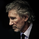# Pivot Points [Floor,DeMark,Woodie,Camarilla,Fibo] by KrisWaters

3967 views
What is Pivot Points?
Pivot points are used by traders in equity and commodity exchanges. They're calculated based on the high, low, and closing prices of previous trading sessions, and they're used to predict support and resistance levels in the current or upcoming session. These support and resistance levels can be used by traders to determine entry and exit points, both for stop-losses and profit taking.

5 in 1 Pivot Point Indicator

Floor Pivot Points:
The floor pivot points are the most basic and popular type of pivots . The pivot point is interpreted as the primary support/resistance level - the point at which the main trend is determined. First-third level resistance and support points serve as additional indicators of possible trend reversal or continuation.

Woodie Pivot Points:
Woodie's pivot points are similar to floor pivot points , the difference being is that more weight is given to the Close price of the previous period.

Camarilla Pivot Points:
Camarilla pivot points are a set of eight very probable levels which resemble support and resistance values for a current trend. The most important is that these pivot points work for all traders and help in setting the right stop-loss and profit-target orders.

Tom DeMark's Pivot Points:
Another popular method of calculating the pivots to forecast the future of the trend is Tom DeMark's pivot points , which are not pivot points exactly, but are the predicted lows and highs of the period.

Fibonacci Pivot Points:
Fibonacci pivot point levels are determined by first calculating the floor pivot points . Next, multiply the previous day's range with its corresponding Fibonacci level. Most traders use the 38.2%, 61.8% and 100% retracements in their calculations. Finally, add or subtract the figures you get to the pivot point and you've got your Fibonacci pivot point levels!

Be careful:
By default, pivot points are determined for the selected timeframe. The resolution input value should be at least the timeframe in used. The resolution value larger than the selected timeframe should not be selected. Otherwise, correct data cannot be displayed.

Correct use: If the selected timeframe is 4h, resolution input variable can be select "4h, 1 day, 1 week or 1 month"
Release Notes: Added the Tom DeMark's Pivot Point.EDGEmtd
@EDGEmtd, ok I added the Tom DeMark's Pivot Point. You can see it now.EDGEmtd
@EDGEmtd, In my research I get that Tom DeMark's has not a pivot point.

According the babypips website: "Another popular method of calculating the pivots to forecast the future of the trend is Tom DeMark's pivot points, which are not pivot points exactly, but are the predicted lows and highs of the period."